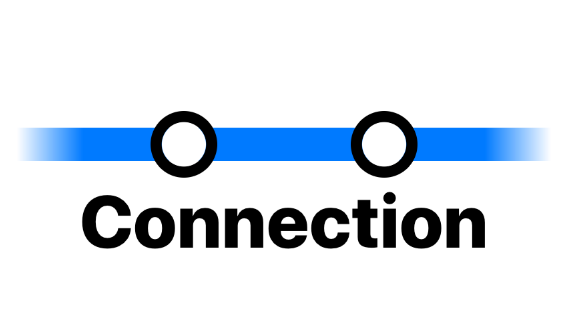/ Miscellaneous## Connection

a Swift path-finding library. Its primary goal is to extend Apple's GameplayKit framework.

## Features

• [x] Weighted connections.
• [x] Total path weight.
• [x] Associated values support.
• [x] Find the shortest path between multiple origins and destinations.

## Usage

Connection defines two new generic classes: `Node` and `Graph`, which are, respectively, `GKGraphNode` and `GKGraph` counterparts.

``````import Connection

// Create nodes.
let nodeA = Node(value: "A")
let nodeB = Node(value: "B")
let nodeC = Node(value: "C")

// Make connections.
nodeA.addConnection(to: nodeB, bidirectional: false, weight: 1)
nodeB.addConnection(to: nodeC, bidirectional: true, weight: 2)

// Create graph.
let graph = Graph([nodeA, nodeB, nodeC])

// Find path.
let shortestAtoCPath = graph.findPath(from: nodeA, to: nodeC)

print(shortestAtoCPath) // ["A", "B", "C"]
``````

You can find many more examples in the `Tests` folder.

## Installation

Connection is distributed using the Swift Package Manager. To install it into a project, follow this tutorial and use this repository URL: `https://github.com/zntfdr/Connection.git`.